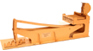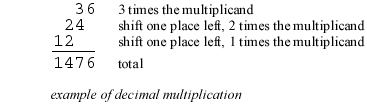The ternary calculating machine of Thomas Fowler home - about - contact Thomas Fowler Balanced ternary arithmetic Fowler's binary and ternary tables DeMorgan's description The reconstruction About the machine Photo gallery References and links Acknowledgements Wanted Balanced ternary arithmetic Balanced ternary, also called signed ternary, is a variation of Base-3 notation in which each digit can be expressed as either a negative one, a zero or a positive one, written as –, 0 and + by Fowler. The chart at the right shows decimal (Base-10) values from -16 to +16, and their corresponding values in Base-3 and balanced ternary. Arithmetic in balanced ternary is much like that for any alternate base, with the exception that there could be both negative and positive digits in the expression of a number. This means that in addition and subtraction there might be both positive and negative carries required to adjacent digits.From the chart, it can be observed that the negative value of any balanced ternary number can be obtained by simply replacing every + with a – and vice versa. It can be easier to subtract a number by inverting the + and – digits and then using normal addition (this technique is used in several of the examples below). Another feature of balanced ternary is that it can express negative values as easily as positive ones, without the need for a leading negative sign as with decimal numbers. Multiplication in balanced ternary is done in a very similar fashion to decimal multiplication. Using the standard practice of multiplying one factor (the multiplicand) by the other factor (the multiplier) one digit at a time, the decimal equation (12 times 123 equals 1476) is calculated in decimal as follows:Similarly in balanced ternary, multiplication is also done one digit at a time. The choice at each digit is much simpler: either add (if the digit is a “+”), subtract (if the digit is a “-”) or ignore (if the digit is a “0”). For example, the decimal equation (2 times 8 equals 16) becomes (+- times +0- equals +--+) in balanced ternary and is calculated as follows:Division in balanced ternary can be calculated in the same manner as decimal long division, but becomes slightly more complex with the possibility of both positive and negative digits in the quotient. For comparison purposes, the following is a simple decimal equation (280 divided by 8 equals 35) calculated by decimal long division:At each stage in decimal long division, the intermediate result is compared to the divisor. The next digit of the quotient is the maximum number of times the divisor goes into the intermediate result without causing the following intermediate result to be negative. Unlike decimal long division, in balanced ternary the intermediate results are allowed to be negative. The next digit of the quotient is either + or – depending on which value will reduce the number of digits in the following intermediate result. The same decimal equation (280 divided by 8 equals 35) becomes (+0++0+ divided by +0- equals ++0-)in balanced ternary and is calculated as follows: